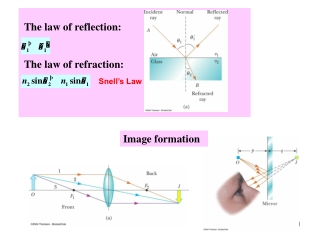DownloadDownload PresentationThe law of reflection:

# The law of reflection:

Download Presentation## The law of reflection:

- - - - - - - - - - - - - - - - - - - - - - - - - - - E N D - - - - - - - - - - - - - - - - - - - - - - - - - - -
##### Presentation Transcript

1. The law of reflection: The law of refraction: Snell’s Law Image formation

2. Reading: Chapter 23

3. Chapter 23 Propagation of Light - Ray Optics

4. Propagation of Light – Ray (Geometric) Optics • Main assumption: • light travels in a straight-line path in a uniform medium and • changes its direction when it meets the surface of a • different medium or • if the optical properties of the medium are nonuniform The rays (directions of propagation) are straight lines perpendicular to the wave fronts The above assumption is valid only when the size of the barrier (or the size of the media) is much larger than the wavelength of light Main Question of Ray Optics: What happens to light at the boundary between two media?

5. Propagation of Light - Ray Optics What happens to light at the boundary between two media? • The light can be • reflected or • refracted (transmitted)

6. Reflection of Light The law of reflection: The angle of reflection is equal to the angle of incidence The incident ray, the reflected ray and the normal are all in the same plane

7. Reflection of Light Specular reflection (reflection from a smooth surface) – example: mirrors Diffuse reflection (reflection from a rough surface)

8. Example: Multiple Reflection (1) The incident ray strikes the first mirror (2) The reflected ray is directed toward the second mirror (3) There is a second reflection from the second mirror (3) (2) (1)

9. Propagation of Light - Ray Optics What happens to light at the boundary between two media? • The light can be • reflected or • refracted (transmitted)

10. Refraction – Snell’s Law • The incident ray, the refracted ray, and the normal all lie on the same plane • The angle of refraction is related to the angle of incidence as • v1 is the speed of the light in the first medium and v2 is its speed in the second Since and , we get , or Snell’s Law index of refraction

11. Light is refracted into a crown glass slab Θ1 = 30.0o , Θ2 = ? n1= 1.0 and n2 = 1.52 n1sin Θ1= n2 sin Θ2then Θ2 = sin-1[(n1 / n2) sin Θ1]= 19.2o Snell’s Law: Example

12. Refraction in a Prism

13. Variation of Index of Refraction with Wavelength Snell’s Law • The index of refraction depends on the wavelength (frequency) • It generally decreases with increasing wavelength So

14. Refraction in a Prism • Since all the colors have different angles of deviation, white light will spread out into a spectrum • Violet deviates the most • Red deviates the least • The remaining colors are in between

15. The Rainbow • The rays leave the drop at various angles • The angle between the white light and the most intense violet ray is 40° • The angle between the white light and the most intense red ray is 42° Water drop

16. Total Internal Reflection

17. Possible Beam Directions: Total Internal Reflection Snell’s Law • Possible directions of the beam are indicated by rays numbered 1 through 5 • The refracted rays are bent away ( ) from the normal since • For ray 4 we have the corresponding angle of incidence can be found from the condition ( )

18. Total Internal Reflection: Critical Angle Snell’s Law • Critical angle: • IMPORTANT: All the rays with will be totally reflected, because if then we get from Snell’ Law This is impossible Example: What is for glass-air boundary? then

19. Total Internal Reflection: Application Fiber Optics Total Internal Reflection ( ) • Plastic or glass rods are used to “pipe” light from one place to another • Applications include: • medical use of fiber optic cables for diagnosis and correction of medical problems • Telecommunications

20. - The speed of light in the medium The law of reflection: The law of refraction: Snell’s Law Total Internal Reflection